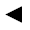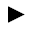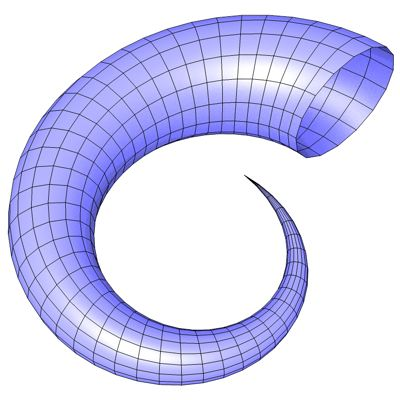﻿﻿﻿ math handbook calculator - Fractional Calculus Computer Algebra System software+++=﻿[back]

## 3.44. horn

[next]The horn [ 8 ] is represented by the following equations.

 x = (a + u cos(v)) sin(b pi u) 3-147 y = (a + u cos(v)) cos(b pi u) + cu 3-148 z = u sin(v) 3-149

The constants a, b and c determine the appearance of the figure.

To represent the area, the two parameters u and v can assume the following values ​​(domain of definition), for example.

 u is an element from the set of numbers [0, 1] v is an element from the number set [-pi, pi]

The domain of definition cannot be changed in the plugin.
The plugin creates an optimized mesh without duplicate points and unconnected polygons.Fig. 48